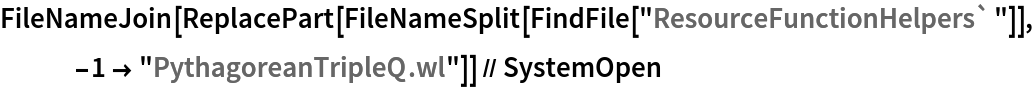Function Repository Resource:

# PythagoreanTripleQ

Determine whether a triple of integers constitutes a Pythagorean triple

Contributed by: Jordan Hasler, Wolfram|Alpha Math Team
 ResourceFunction["PythagoreanTripleQ"][{a,b,c}] determines whether the triple of integers {a,b,c} satisfies a2+b2=c2.

## Details

The option "UnorderedTriple" determines whether to treat the input as ordered and defaults to False. When set to True, ResourceFunction["PythagoreanTripleQ"] returns True if any ordering of the triples satisfies a2+b2=c2.

## Examples

### Basic Examples (2)

The ordered triple {3,4,5} is a Pythagorean triple:

 In:=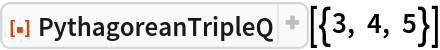Out=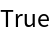{2,3,4} is not a Pythagorean triple:

 In:=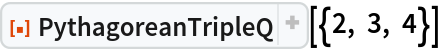Out=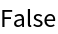### Options (2)

{5,4,3} is not a Pythagorean triple:

 In:=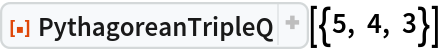Out=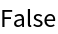Specify that the triple is unordered to determine if any ordering of the numbers is a Pythagorean triple:

 In:=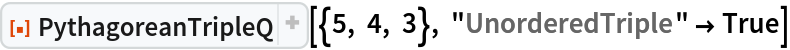Out=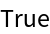### Properties and Relations (2)

To determine whether any ordering of potentially noninteger side lengths forms a right triangle, use RightTriangleQ:

 In:=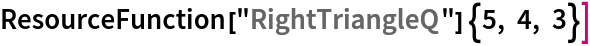Out=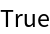In:=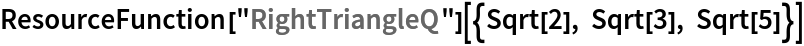Out=The integers {5,4,3} can form a right triangle when considered as edge lengths, but do not form a Pythagorean triple due to their ordering:

 In:=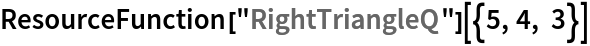Out=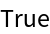In:=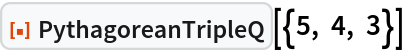Out=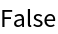## Publisher

Wolfram|Alpha Math Team

## Version History

• 2.0.0 – 23 March 2023
• 1.0.1 – 29 March 2021
• 1.0.0 – 18 September 2020

## Author Notes

To view the full source code for PythagoreanTripleQ, run the following code:

 In:=